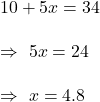## Parker wants to ride his bicycle 34 miles this week. He has already ridden 10 miles. If he rides for 5 more days, how many miles did he ride

Question

Parker wants to ride his bicycle 34 miles this week. He has already ridden 10 miles. If he rides for 5 more days, how many miles did he ride each day? Define the variable and write an equation.

in progress 0
2 months 2021-07-29T18:23:04+00:00 1 Answers 2 views 0

1. Answer: He rode 4.8 miles each day.

Variable: x = Constant distance distance traveledd each day.

Equation : 10+5x=34

Step-by-step explanation:

Total distance needs to travel = 34 miles

Distance already traveled = 10 miles

He will ride bicycle for 5  more days.

Let x = Constant distance distance traveledd each day.

Total distance = 10+5x

i.e.Hence, he rode 4.8 miles each day.